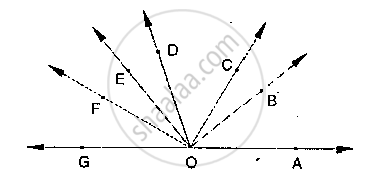# If Below Fig, ∠Aof and ∠Fog Form a Linear Pair.∠Eob = ∠Foc = 90° and ∠Doc = ∠Fog = ∠Aob = 30°(I) Find the Measures of ∠Foe, ∠Cob and ∠Doe.(Ii) Name All the Right Angles. - Mathematics

If below fig, ∠AOF and ∠FOG form a linear pair.∠EOB = ∠FOC = 90° and ∠DOC = ∠FOG = ∠AOB = 30°
(i) Find the measures of ∠FOE, ∠COB and ∠DOE.
(ii) Name all the right angles.
(iii) Name three pairs of adjacent complementary angles.
(iv) Name three pairs of adjacent supplementary angles.
(v) Name three pairs of adjacent angles.

#### Solution

(i)  ∠FOE = x, ∠DOE = y and ∠BOC = z sat

Since ∠AOF , ∠FOG is Linear pair

⇒∠AOF + 30° = 180°              [∠AOF + ∠FOG = 180° and ∠FOG = 30°]

⇒ ∠AOF = 180° - 30°

⇒ ∠AOF = 150°

⇒ ∠ AOB + ∠BOC + ∠COD + ∠DOE + ∠EOF = 150°

⇒ 30° + z + 30° + y + x = 150°

⇒ x + y + z = 150° - 30° - 30°

⇒ x + y + z = 90°                          .....(1)

Now ∠FOC = 90°

⇒ ∠FOE + ∠EOD + ∠DOC = 90°

⇒ x + y + 30° = 90°

⇒ x + y = 90° - 30°

⇒ x + y = 60°                              .....(2)

Substituting (2) in (1)

x + y + z = 90°

60 + z = 90° Þ z = 90° - 60° = 30°

i.e., ∠BOC = 30°

Given ∠BOE = 90°

∠BOC + ∠COD + ∠DOE = 90°

⇒ 30° + 30° + ∠DOE = 90°

⇒ ∠DOE = 90° - 60° = 30°

∴ ∠DOE = x = 30°

Now, also we have

x + y = 60°

⇒ y = 60° - x = 60° - 30° = 30°

∠FOE = 30

(ii) Right angles are

∠DOG, ∠COF , ∠BOF , ∠AOD

(iii) Three pairs of adjacent complementary angles are

∠AOB, ∠BOD;

∠AOC, ∠COD;

∠BOC, ∠COE

(iv) Three pairs of adjacent supplementary angles are

∠AOB, ∠BOG;

∠AOC, ∠COG;

∠AOD, ∠DOG.

(v) Three pairs of adjacent angles

∠BOC, ∠COD;

∠COD, ∠DOE;

∠DOE, ∠EOF ,

Concept: Pairs of Angles
Is there an error in this question or solution?
Chapter 10: Lines and Angles - Exercise 10.2 [Page 16]

#### APPEARS IN

RD Sharma Mathematics for Class 9
Chapter 10 Lines and Angles
Exercise 10.2 | Q 18 | Page 16

Share